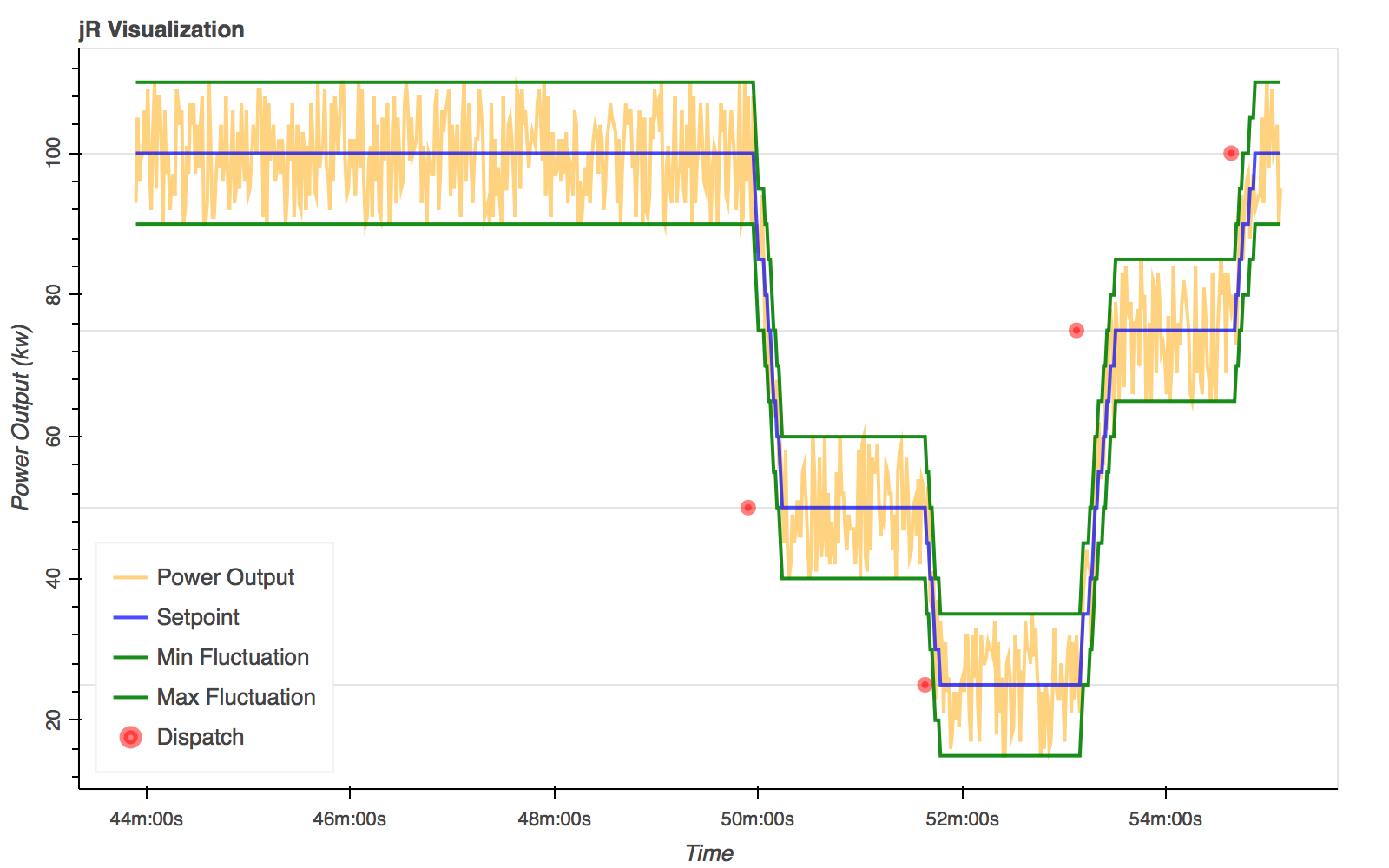# Timeseries data generation with PyPoSim

Inspired by one of our projects at work, I decided some time ago to write a small simulator that generates noisy power output of a powerplant.

The code can be found in the PyPoSim Github Repo

Using this project, let’s generate some timeseries data, store it to a CSV and visualize it.

## The basics

The first thing to do is to start PyPoSim.

``````mkdir tslog # folder where the timeseries data gets stored by default
python PyPoSim.py
``````

Make sure to have all dependencies installed using pip.

``````pip install -r requirements.txt
``````

One plant is already existing by default with the ID `jR`. Doing a curl to request the masterdata for that plant will give us some more detailed information about it:

``````curl localhost:5000/jR
``````
``````{"fluctuationInPercentage": 10, "rampInSeconds": 5, "capacity": 100, "uid": "jR", "name": "Default"}
``````
• `fluctuationInPercentage` is the fluctuation running around the power in percent
• `capacity` is the power-level that this plant is able to generate at max. The output fluctuates around this value.
• `ramp` is the factor the plant can move up and down to certain outputs per second in KW

## Pythonize the basics

The masterdata information is important to check the behaviour of the plant. As we use the generated CSV for the visualization we can hardcode the important things.

``````capacity = 100
fluctuation = 10.0/100.0
``````

After a couple of minutes playing around with the UI and requesting the plant to change it’s output (`dispatch`), the following CSV got generated.

We can easily read it using Pandas, a data analysis library for Python. If not already done, install it via pip.

``````pip install pandas
``````

Afterwards it can be used to read the CSV into a dataframe.

``````import Pandas as pd
``````

The data is represented as a dataframe, an array structure with the same schema as the CSV. The newly generated index is not needed as we use the timestamp for this purpose.

``````df = df.set_index(pd.DatetimeIndex(df['ts']).round("1S"))
ts = df.drop(['ts'], axis=1)
``````

## Filter for the right information

From now on the dataframe can be wrangled until everything is seperated nicely. The CSV schema is generic but narrow, which leads to a lot of rows. From there on we start separating different metrics (e.g. sensornames like power_output) into individual timeseries.

PyPoSim generates datapoints (metric + timestamp) for 3 metrics, `power_output` `setpoint` and `dispatch`. As the name infers, `power_output`is the currently generated power. `setpoint`is the internal target value that the plant is supposed to generate as accurately as possible given the fluctuation rate. `dispatch`is an event which sets a new setpoint

``````power = ts[ts['metric']=="power_output"]
setpoint = ts[ts['metric']=="setpoint"]
setpoint['min_fluctuation'] = setpoint['value']-(capacity*fluctuation)
setpoint['max_fluctuation'] = setpoint['value']+(capacity*fluctuation)
dispatch = ts[ts['metric']=="dispatch"]
``````

The setpoint dataframe get’s also enriched by a corridor which indicates if the generation is in it’s fluctuation boundaries. In a real-world scenario this can be exceeded either if a plant is not targeting it’s setpoint correctly by whatever cause, or it is not as accurate as assumed or parts are broken which lead to certain patterns in output generation or or or…

## Let’s see what comes out

`Bokeh` will be used to generate a plot that shows us the plant behaviour over time.

First of all `bokeh` has to be installed:

``````pip install bokeh
``````

After that the plot can be created and each dataframe can be added to it. `power_output`and `setpoint`will be added as lines, the discrete `dispatch` events are added as scatters.

``````from bokeh.plotting import figure, show
from bokeh.io import output_file
from bokeh.charts import Scatter, TimeSeries
from bokeh.models.formatters import DatetimeTickFormatter, NumeralTickFormatter
from bokeh.models.tickers import SingleIntervalTicker

output_file("jr.html")

p = figure(title="jR Visualization", plot_width=800, plot_height=500, x_axis_type="datetime")
p.line(power.index, power['value'], color='orange', alpha=0.5, line_width=2, legend="Power Output")
p.line(setpoint.index, setpoint['value'], color='blue', alpha=0.7, line_width=2, legend="Setpoint")
p.line(setpoint.index, setpoint['min_fluctuation'], color='green', alpha=0.9, line_width=2, legend="Min Fluctuation")
p.line(setpoint.index, setpoint['max_fluctuation'], color='green', alpha=0.9, line_width=2, legend="Max Fluctuation")
p.scatter(dispatch.index, dispatch['value'], color='red', alpha=0.5, line_width=5, legend="Dispatch")
p.legend.location = "bottom_left"

p.xaxis.axis_label = "Time"
p.xaxis.formatter = DatetimeTickFormatter(minutes=['%Mm:%Ss'],minsec=['%Mm:%Ss'])
p.yaxis.axis_label = "Power Output (kw)"
p.yaxis.major_label_orientation = "vertical"

p.xgrid.grid_line_color = None
p.grid.ticker = SingleIntervalTicker(interval=25)

show(p)
``````

What we can see is that the plant received 4 dispatch commands within the visualized timeframe. The first one demands it to ramp to 50kw, the second one to 25kw, then 75kw and at the end back to 100kw.Furthermore we can see how the power output follows the given setpoint and stays in it’s boundaries (which makes sense as PyPoSim has currently no misbehaviour programmed in it’s model).

## Next steps

From that point the are a variety of steps to go further. Either the `Simpleplant` model in PyPoSim can be extended to simulate misbehaviour that has to be detected or using Kafka `jR` could be visualized and observed in real-time which leads to a whole new set of complexities to be solved like performant javascript charting.